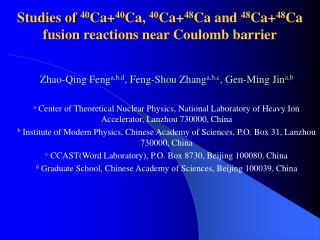DownloadDownload PresentationStudies of 40 Ca+ 40 Ca, 40 Ca+ 48 Ca and 48 Ca+ 48 Ca fusion reactions near Coulomb barrier

# Studies of 40 Ca+ 40 Ca, 40 Ca+ 48 Ca and 48 Ca+ 48 Ca fusion reactions near Coulomb barrier

Télécharger la présentation## Studies of 40 Ca+ 40 Ca, 40 Ca+ 48 Ca and 48 Ca+ 48 Ca fusion reactions near Coulomb barrier

- - - - - - - - - - - - - - - - - - - - - - - - - - - E N D - - - - - - - - - - - - - - - - - - - - - - - - - - -
##### Presentation Transcript

1. Studies of 40Ca+40Ca, 40Ca+48Ca and 48Ca+48Ca fusion reactions near Coulomb barrier Zhao-Qing Fenga,b,d, Feng-Shou Zhanga,b,c, Gen-Ming Jina,b a Center of Theoretical Nuclear Physics, National Laboratory of Heavy Ion Accelerator, Lanzhou 730000, China b Institute of Modern Physics, Chinese Academy of Sciences, P.O. Box 31, Lanzhou 730000, China c CCAST(Word Laboratory), P.O. Box 8730, Beijing 100080, China d Graduate School, Chinese Academy of Sciences, Beijing 100039, China

2. 1. Introduction • Recently, studies on the synthesis of superheavy elements is a hot object. Theoretically, the only existence of the heaviest elements with Z>102 is due to quantal shell effects. The properties of SHEs were studied theoretically using Strutinsky method. The dynamical process of the formation of SHEs is not understood well enough. Microscopic transport theory such as isospin dependent molecular dynamics (IQMD) model or isospin dependent Boltzmann-Uehling-Uhlenbeck (IBUU) model is a suitable model for describing the dynamical process of the SHE formation, which has been used successfully for studying isospin effects of heavy ion collisions at intermediate energies.

3. 2. Improvements of the IQMD model In IQMD, the effective interaction potential energy is composed of the Coulomb interaction potential energy and the local interaction potential energy: • The Coulomb interaction potential energy is expressed as:

4. The above terms can be written as • where rij is given by

5. The tiz is the z-th component of the isospin degree of freedom for the i-th nucleon, which is equal to 1 and –1 for proton and neutron, respectively. The symmetry energy term has included the surface-symmetry energy term which is proposed by Wang Ning et al. (N. Wang, Z. X. Li, X. Z. Wu, et al., Phys. Rev. C 69 (2004) 034608). • The time evolutions of the nucleons in the system under the self-consistently generated mean-field is governed by Hamiltonian equations of motion, which are derived from the time dependent variational principle and is expressed as

6. The surface term of the system is improved by switch function method which collects the surface energies of projectile and target with the one of compound nucleus. The switch function is expressed as • where R is the distance of the centers between projectile and target, Rup and Rlow are the distance between centers at

7. initial time and final time at which the compound nucleus is formed, respectively. Here, the two parameters are set to be 20 fm and 0 fm, respectively, if R is larger than Rup ,then we set R equal to Rup. The parameters C0, C1, C2, C3, C4 and C5are taken to be 0, 0, 0, 10, -15 and 6, respectively, which assure the continuity of the surface energy and its first derivative. Thus, the surface energy of the system is written as • where Uisurf denotes the surface energy term of the i-th system.

8. As we know that shell effect is the diversity of shell model (shell structure) and macroscopic model (bulk property). Thus, the shell correction energy can be obtained from the variance of shell levels and uniformed levels, which is written as • where the factor 2 is the degeneracy of the deformed levels (Nilsson levels), the smooth level density is given by

9. Here g is Gaussian distribution width, which is usually taken to be 1.2, is equal to 41A-1/3. ei is the i-th level which is calculated by DTCSM. F((e-ei)/ g) is the correction function which keeps the long-range variation over energies unchanged, in the calculation, we select 3-order Laguerre polynomial. • From the nucleon number N, we can obtain the Fermi energy, which is expressed as • However, it is difficult to directly impose the force on each nucleon derived from the shell correction energy Eshell. As an approximative treatment, we can write the shell correction energy in the model as

10. Derivating the equation, we can easily obtain the force derived from the shell correction energy as • The ordering of filling in the levels is taken according to angular momentum size as well as single nucleon energy (for the same angular momentum). ri ,R and are coordinate of the center of mass for i-th nucleon, nucleus radius and dispersion width, respectively, for R and a, we take 1.2A-1/3fm and 0.55 fm respectively, where A is the total nucleon number.

11. 3. Results and discussions

12. Thanks a lot for attendance !